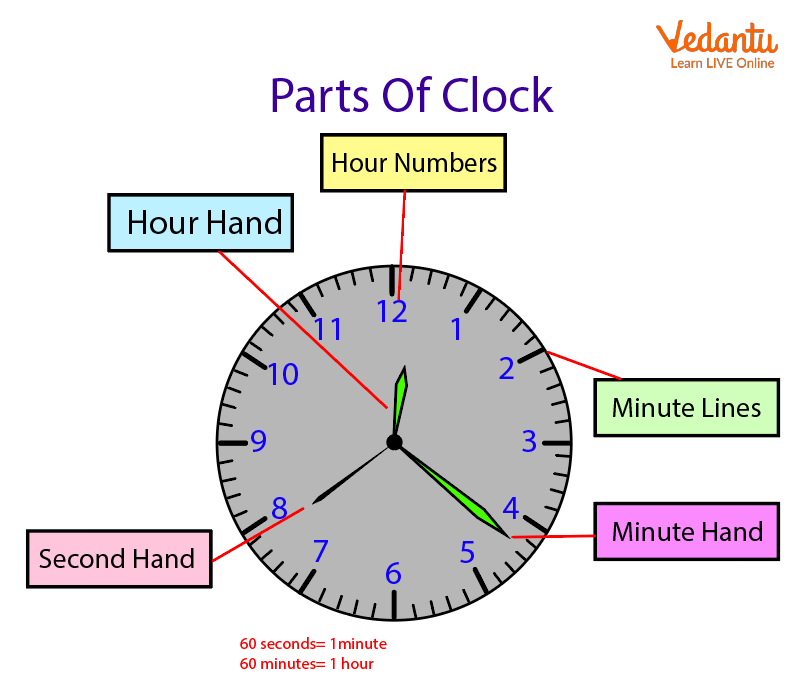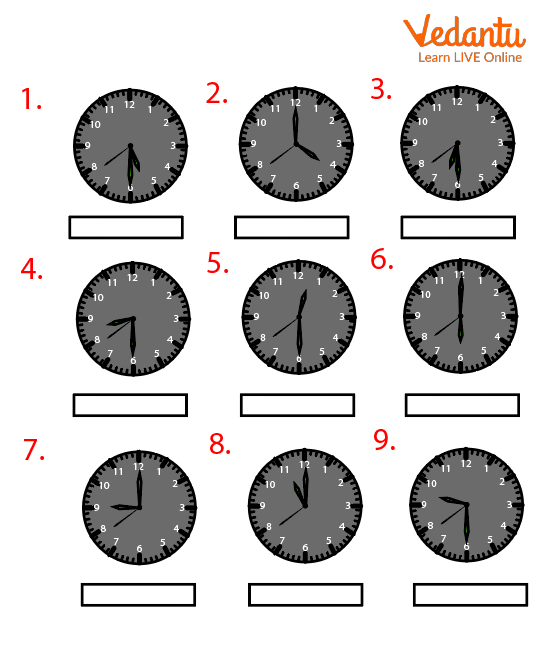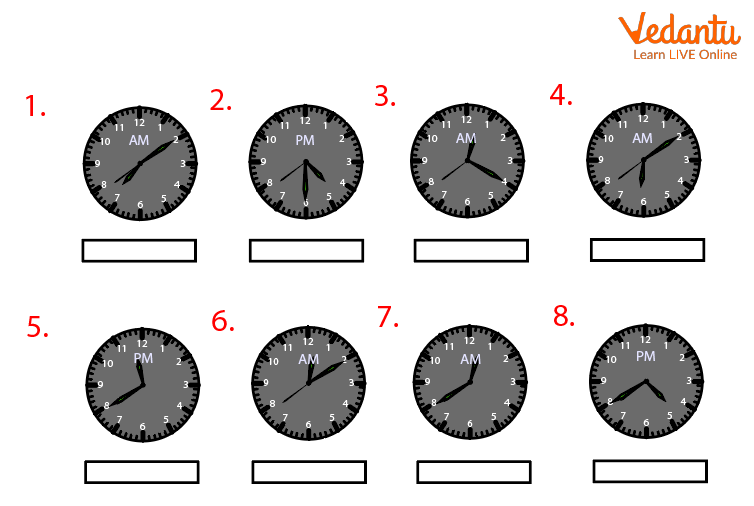Courses
Courses for Kids
Free study material
Offline Centres
MoreLast updated date: 01st Dec 2023
Total views: 103.8k
Views today: 3.03k## Time Reading on a Clock

To determine the time, you can carefully study clocks or quickly glance at the hour hand. Most clocks include hands that indicate the minutes or hours, and each hand can display the current hour in either analog or digital format (an unusual type).

Anything that moves constantly is an analog, therefore the big round clock in your classroom with three sticks spinning around is called an analog clock.

When a typical analog clock shows the time 8:22 and seconds, it could mean. 8:22 a.m or you can't tell from an analog clock if the time is morning (a.m.) or afternoon (p.m.).

Most analog clocks will have three sticks mounted within the center that spins around the clock to tell you the time. These are called clock hands.

Terms of ClockParts of a Clock

### Hour Hand

The little hand is the hour hand. When the little hand is between two numbers, it tells you we are between those two hours. The shorthand or little hand makes one full rotation around the dial in hours.

For example: In the above image the time is 12:22:38. The hand which is near 12 is an hour hand showing 12 hours.

### Minute Hand

The longer hand on the clock is named the minute hand. The long hand or big hand moves around the circular dial one time in one hour.

For example: In the above image the time is 12:22:38. The hand which is near 4 is a minute hand showing 22 minutes.

### Second Hand

The very thin hand that's usually just as long as the minute hand is the second hand. It moves just one occasion around the dial in one minute and indicates the seconds of each minute.

For example: In the above image the time is 12:22:38. The hand which is near 8 is a second hand showing 38 seconds.

### Analog Clock Face

An analog clock or watch is one that uses hands that move and numbers from 1 to 12 to indicate the hours to display the time. 24 hours make up a day. All 24 hours of the day may be identified by a clock with marks from 1 to 12. Roman Numerals (I, II, III, etc.) or no numbers at all can be seen on certain clocks! To tell the time, random analog clocks employ angles. They feature hands that move around the face of the clock.

### How to Read an Analog Clock

To read an analog clock, start with the hours. Examine the shortest hand only; this is the hour hand.

• The little hand will point directly at a number only 12 times in 12 hours. Most of the time, you'll see it pointing between two numbers.

• When the little hand is pointed directly at a clock number, you recognize it is exactly that hour. When the little hand is in between two hours, the amount behind the hand is the current hour.

For example, if the hand is past 1, then we are at a time between 1 o’clock and 2 o’clock. If the hand is past 3, then we've passed 3 o’clock and are approaching 4 o’clock.

Next, we determine the minutes. When measuring minutes or seconds, we've to multiply the printed numbers times 5:

• \[1 = 5\]

• \[2 = 10\]

• \[3 = 15\]

• \[4 = 20\]

• \[5 = 25\]

• \[6 = 30\]

• \[7 = 35\]

• \[8 = 40\]

• \[9 = 45\]

• \[10 = 50\]

• \[11 = 55\]

• \[12 = 60\]

When the big hand is pointing to the 1, it indicates 5 minutes past the present hour. If you divide the dial into four quarters, when the big hand is pointing at the 12, it's a new hour. When it points at the 3, we are past the hour.

When the big hand points at the 6, it's two-quarters past, but we are saying half past the hour because this minute hand is halfway around the dial. This is often equal to one-half hour of elapsed time.

When it's pointing to the first little marker between the 1 and the 2, it indicates that it's 6 minutes past the current hour.

The second user works the same way. It just indicates seconds rather than minutes, and when it's made one full rotation around the clock, it indicates one minute has elapsed.

## How to Read the Time of the Hour

To tell the time of the hour, say the hour by number and add o’clock to the top of it. Let’s explore the way to tell time on the hour, using the examples below starting from 1-12.

Time Written in English

\[1:00\] It's one o'clock

\[2:00\] It's two o'clock

\[3:00\] It's three o'clock

\[4:00\] It's four o'clock

\[5:00\] It's five o'clock

\[6:00\] It's six o'clock

\[7:00\] It's seven o'clock

### Exact timing

Time Written in English

\[1:18\] It’s one hour and eighteen minutes

\[2:23\] It’s two hours and twenty-three minutes.

\[3:47\] It’s three hours and forty-seven minutes.Ans:

1. 5:30

2. 4:00

3. 6:30

4. 8:30

5. 12:30

6. 6:00

7. 9:00

8. 11:00

9. 9:30

## Solved QuestionsAns:

1. 7:10 AM

2. 4:30 PM

3. 12:40 AM

4. 6:10 AM

5. 11:40 PM

6. 12:10 AM

7. 12:40 AM

8. 4:40 PM

## Summary

In this chapter, we have discussed clock timing. An analog clock is nothing but a timekeeping device with numbers around the edge with hands that rotate around to indicate the time. That device is known as a clock. We have studied the terms related to clocks like a hand, hours, minute, etc.